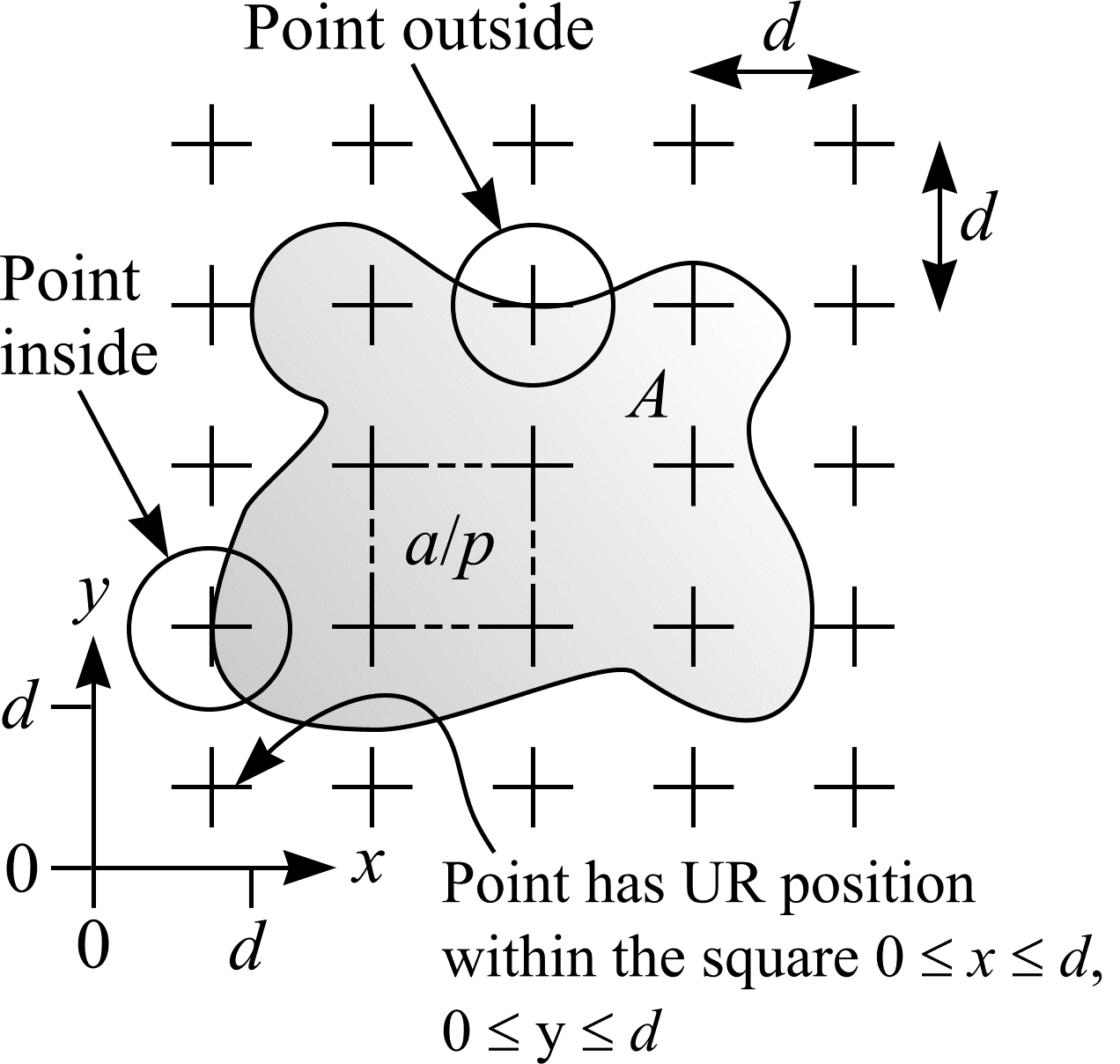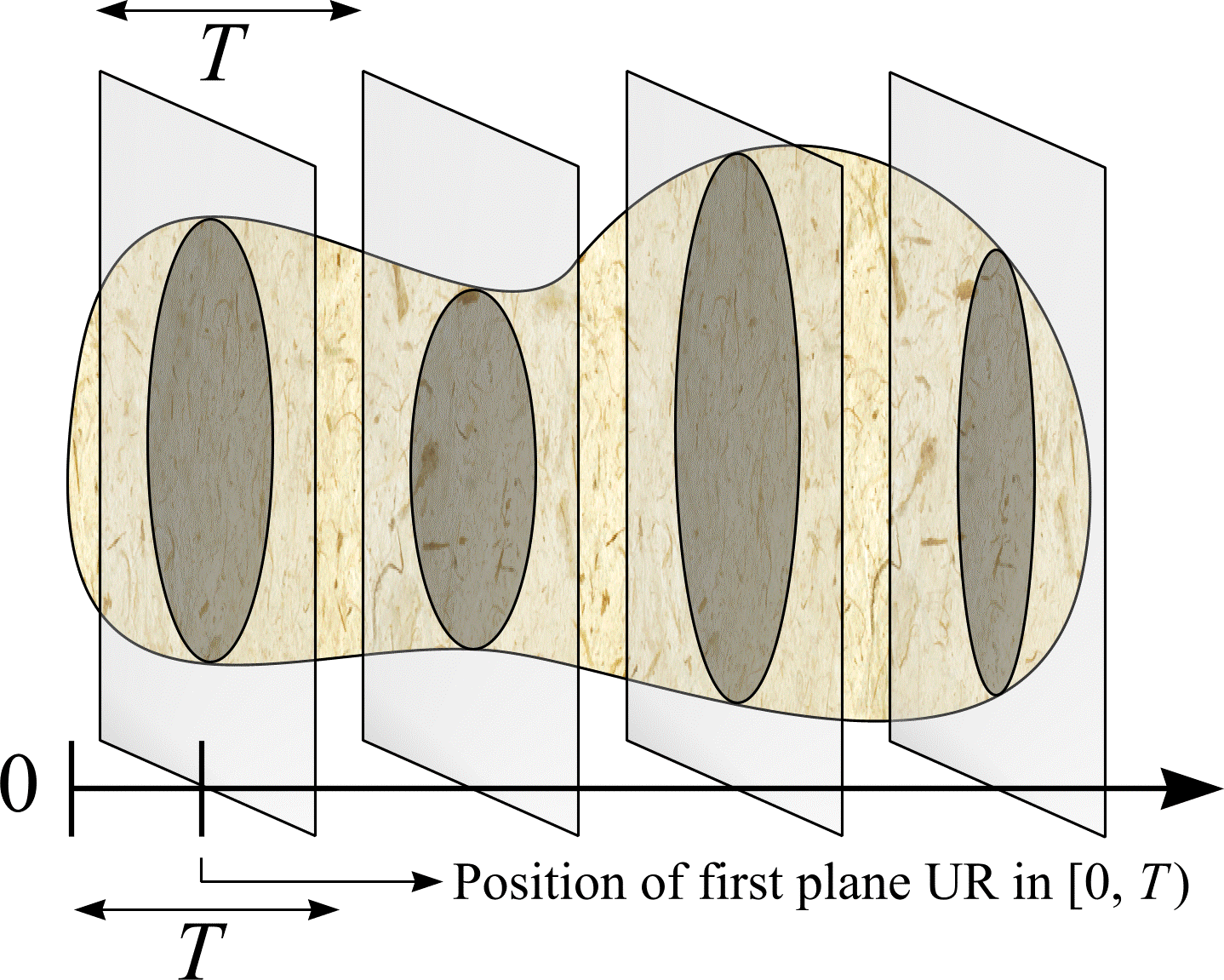# Area and volume

Consider a bounded object in $$\real^2$$. An example is shown in Figure 8. The area, $$A$$, of the object can be efficiently estimated by point counting. Onto the object, overlay a test system of points uniform randomly and count the number of test points hitting the object. The sample space or set of all possible outcomes, $$\Omega$$, is {0 points hit object, 1 point hits object, 2 points hit object, ...}. Let $$P$$ be the random variable $$P(\omega) = \omega$$. If the area per test point is $$a/p$$, then

$$A = \frac{a}{p} \cdot \mathbf{E}P. \tag{25}$$

An estimate of $$A$$ can be obtained from a single throw of the test system of points:

$$\hat{A} = \frac{a}{p} \cdot P. \tag{26}$$Figure 8: Area estimation using a test system of points. The area per point is $$a/p$$. A test point is a zero dimensional probe; to remove the influence of line thickness, Weibel (1979, p.113) defines a test point as the true point of intersection between the upper edge of the horizontal line of a cross, and the right-hand side edge of the vertical line of the cross. In this example, $$P$$ = 9.

Now consider a bounded object in $$\real^3$$. An example is shown in Figure 9. The volume, $$V$$, of the object can be estimated if the object is systematically sampled by plane sections. Fix a convenient axis relative to the object. Next, intersect the object by a Uniform Random (UR) systematic set of parallel planes, a given distance $$T$$ apart, and normal to the chosen axis. Let the random variables $$A_{1}$$, $$A_{2}$$, ..., $$A_{m}$$ denote the successive cross-sectional areas formed by the intersection of the bounded object with the parallel planes. If the random variable $$A_{T}$$ represents the sum of the cross section areas,

$$V = T \cdot \mathbf{E}A_{T}. \tag{27}$$

An estimate of $$V$$ can be obtained from a single throw of the parallel plane test system:

$$\hat{V} = T \cdot \sum_{i=1}^{n} A_{i}. \tag{28}$$

A further approximation of $$V$$ is possible if each cross sectional area is estimated with a uniform random grid of points:

\begin{align} \hat{V} &= T \cdot \sum_{i=1}^{n} A_{i} \\ &= T \cdot \frac{a}{p} \cdot \sum_{i=1}^{n} \mathbf{E}P_{i}, \end{align} \tag{29}

so that

$$\hat{V_{2}} = T \cdot \frac{a}{p} \cdot \sum_{i=1}^{n} P_{i}. \tag{30}$$Figure 9: Volume estimation using the Cavalieri method.

This method of volume estimation is called the Cavalieri method. The method is named after the Italian mathematician Bonaventura Cavalieri (1598-1647), a pupil of Galileo. To apply the Cavalieri method without incurring bias, sections have to be truly planar or infinitely thin. If the distance, $$T$$, between parallel sections varies, then $$T$$ may be replaced with its mean value. There are many examples where the Cavalieri method has been used to estimate volume. Michel and Cruz-Orive (1988) estimated lung volume. Roberts et al. (1993) and (1994) used MRI to estimate, respectively, human body composition and fetal volume.

## References

MICHEL, R. P. and CRUZ-ORIVE, L. M. Application of the Cavalieri principle and vertical sections method to lung: estimation of volume and pleural surface area. J. Microsc., 150, 117-136 (1988).

ROBERTS, N., CRUZ-ORIVE, L. M., REID, M., BRODIE, D., BOURNE, M. and EDWARDS, R. H. T. Unbiased estimation of human body composition by the Cavalieri method using magnetic resonance imaging. J. Microsc., 171, 239-253 (1993).

ROBERTS, N., GARDEN, A. S., CRUZ-ORIVE, L. M., WHITEHOUSE, G. H. and EDWARDS, R. H. T. Estimation of fetal volume by MRI and stereology. The British Journal of Radiology, 67, 1067-1077 (1994).

WEIBEL, E. R. Stereological Methods. Vol. 1: Practical Methods for Biological Morphometry. London - New York - Toronto: Academic Press (1979).